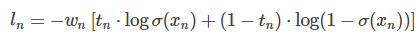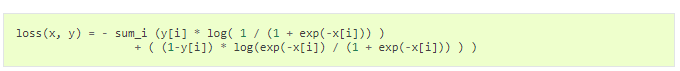# What is the difference between BCEWithLogitsLoss and MultiLabelSoftMarginLoss

I think there is no difference between BCEWithLogitsLoss and MultiLabelSoftMarginLoss.

BCEWithLogitsLoss = One Sigmoid Layer + BCELoss (solved numerically unstable problem)

MultiLabelSoftMargin’s fomula is also same with BCEWithLogitsLoss.

One difference is BCEWithLogitsLoss has a ‘weight’ parameter, MultiLabelSoftMarginLoss no has)

BCEWithLogitsLoss :MultiLabelSoftMarginLoss :The two formula is exactly the same except for the weight value.

10 Likes

You are right. Both loss functions seem to return the same loss values:

``````x = Variable(torch.randn(10, 3))
y = Variable(torch.FloatTensor(10, 3).random_(2))

# double the loss for class 1
class_weight = torch.FloatTensor([1.0, 2.0, 1.0])
# double the loss for last sample
element_weight = torch.FloatTensor([1.0]*9 + [2.0]).view(-1, 1)
element_weight = element_weight.repeat(1, 3)

bce_criterion = nn.BCEWithLogitsLoss(weight=None, reduce=False)
multi_criterion = nn.MultiLabelSoftMarginLoss(weight=None, reduce=False)

bce_criterion_class = nn.BCEWithLogitsLoss(weight=class_weight, reduce=False)
multi_criterion_class = nn.MultiLabelSoftMarginLoss(weight=class_weight, reduce=False)

bce_criterion_element = nn.BCEWithLogitsLoss(weight=element_weight, reduce=False)
multi_criterion_element = nn.MultiLabelSoftMarginLoss(weight=element_weight, reduce=False)

bce_loss = bce_criterion(x, y)
multi_loss = multi_criterion(x, y)

bce_loss_class = bce_criterion_class(x, y)
multi_loss_class = multi_criterion_class(x, y)

bce_loss_element = bce_criterion_element(x, y)
multi_loss_element = multi_criterion_element(x, y)

print(bce_loss - multi_loss)
print(bce_loss_class - multi_loss_class)
print(bce_loss_element - multi_loss_element)
``````
10 Likes

Thank you for your reply, And I confirmed that there was no difference.

But, I wonder why the same functions are separated.

P.S.
what version of pytorch do you use?
my pytorch version is ‘0.3.0 post4’, this version doesn’t have a ‘reduce’ parameter in BCEWithLogitsLoss and MultiLabelSoftMarginLoss.

I’m using `'0.4.0a0+5eefe87'` (compiled a while ago from master).
You could update to `0.3.1`, although the `reduce` argument will still be missing (doc) or compile from master.
You can find the build instructions here.

What’s the plan with these two functions? Will one of them be deleted?

Can you better explain your argument? I do not understand why one of them should not be deleted.

I was referring to BCEWithLogitsLoss and MultiLabelSoftMarginLoss.

up… Can anyone explain which to use?

1 Like

@albanD @smth @apaszke can we please have some clarifications on this? I can verify that both the losses indeed give the same values for the same input.

1 Like

I have the same confusion about the two loss functions.

As far as I understand, BCEWithLogitsLoss is used for Binary Cross Entropy loss and MultiLabelSoftMarginLoss for Multi-Label Cross Entropy loss.

Sure, when you have a binary case both of them will give you the same result. But using BCEWithLogitsLoss for binary classification will make your code more readable and easier to comprehend.

5 Likes